## python内置数据结构字符串

2021/2/8 12:15:08 文章标签: 测试文章如有侵权请发送至邮箱809451989@qq.com投诉后文章立即删除

# 一、字符串的创建和赋值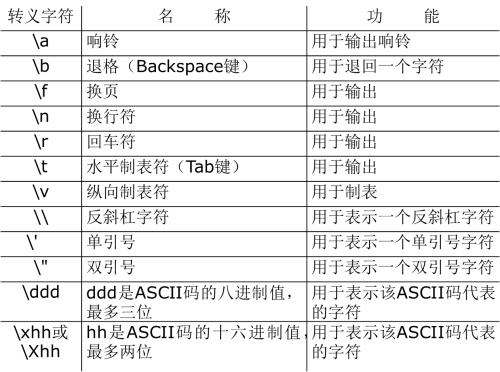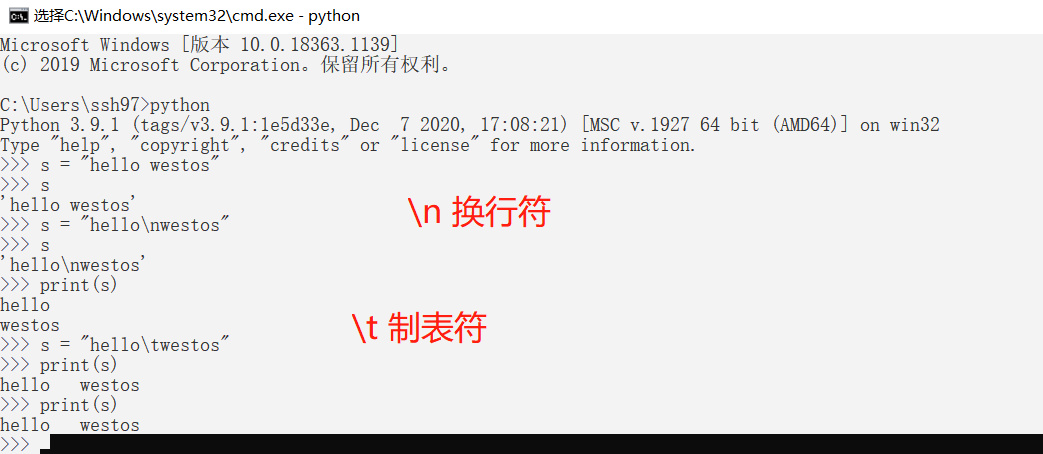# 二、字符串的基本特性

## 1.连接操作符与重复操作符

``````## 1.连接操作符和重复操作符
name = "world"
print ('hello '  + name)
print ("*"*30 + '学生管理系统' + "*"*30)
``````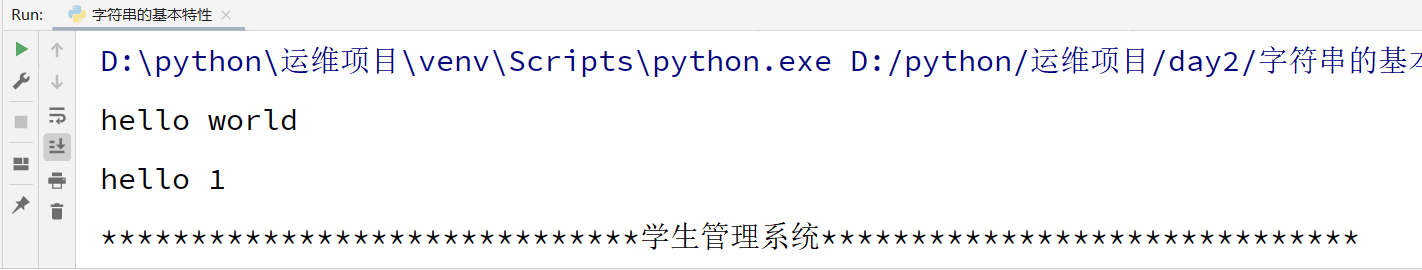Pycharm常用的快捷键:

## 2.成员操作符

``````## 2.成员操作符
s = "hello world"
print('hello' in s)   #True
print('world' not in s) #False
``````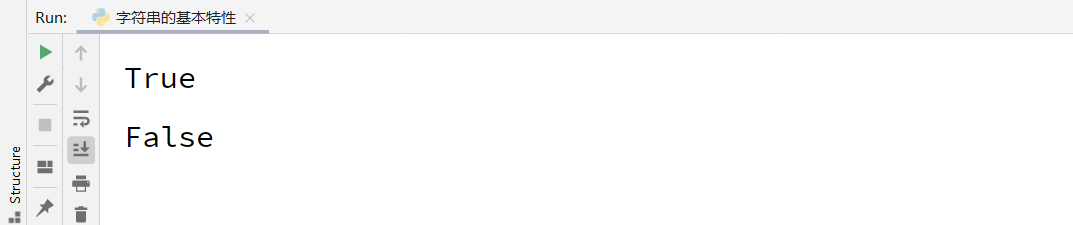## 3.正向索引和反向索引

1. 索引(s[i] )： 获取特定偏移的元素
2. 索引的分类: 正向索引, 反向索引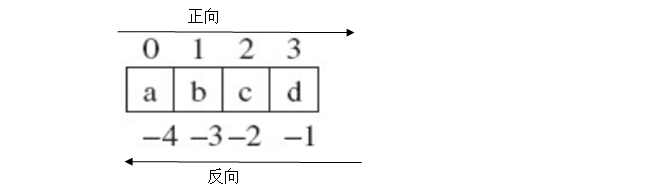``````## 3.正向索引和反向索引
s = 'hello'
print(s)  #'h'
print(s)  #'l'
print(s[-3]) #'l'
``````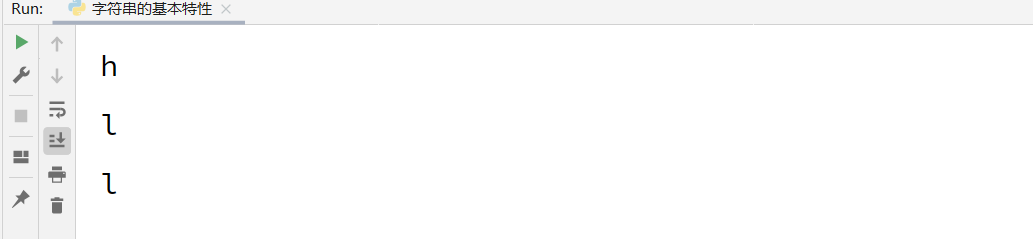## 4.切片

``````## 4.切片
"""

s[start:end:step]
s[:end]:
s[start:]:
"""
s = 'hello world'
print(s[1:3])  # 从1开始到3-1结束
print(s[:3])  # 从0开始到3-1 结束
print(s[:5])  # 前五个字符
print(s[3:])  # 从第3个开始，到结束所有字符
print(s[::])  # 拷贝字符串  拿出所有字符串
print(s[::-1])  #倒序
``````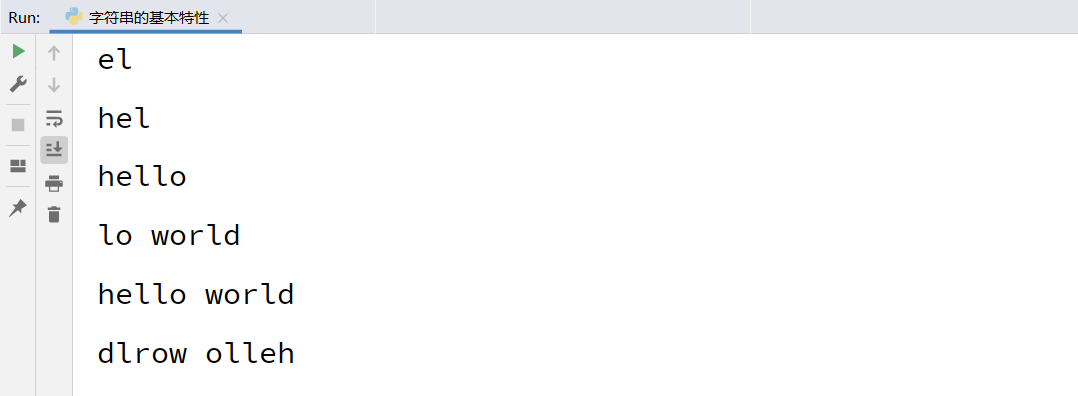## 5. 可迭代对象for循环访问

``````## 5. 可迭代对象for循环访问
s = 'hello'
count = 0
for item in s:
count +=1
print(f'第{count}字符是{item}')
``````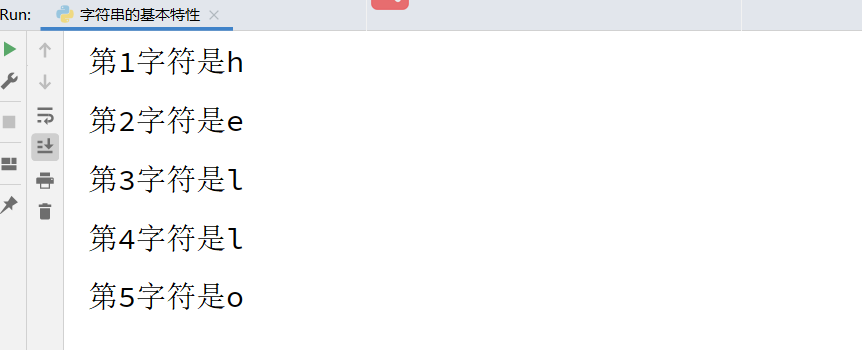``````"""

"""
s = input("输入字符串：")
result = "回文字符串" if s == s[::-1] else "不是回文字符串"
print(s + result)
``````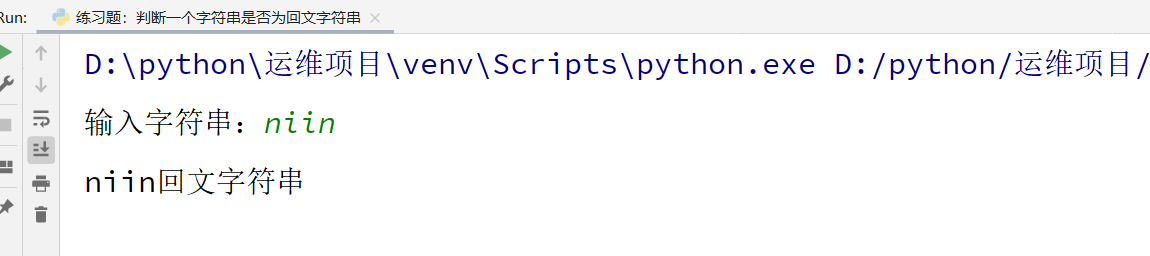# 三、字符串的内建方法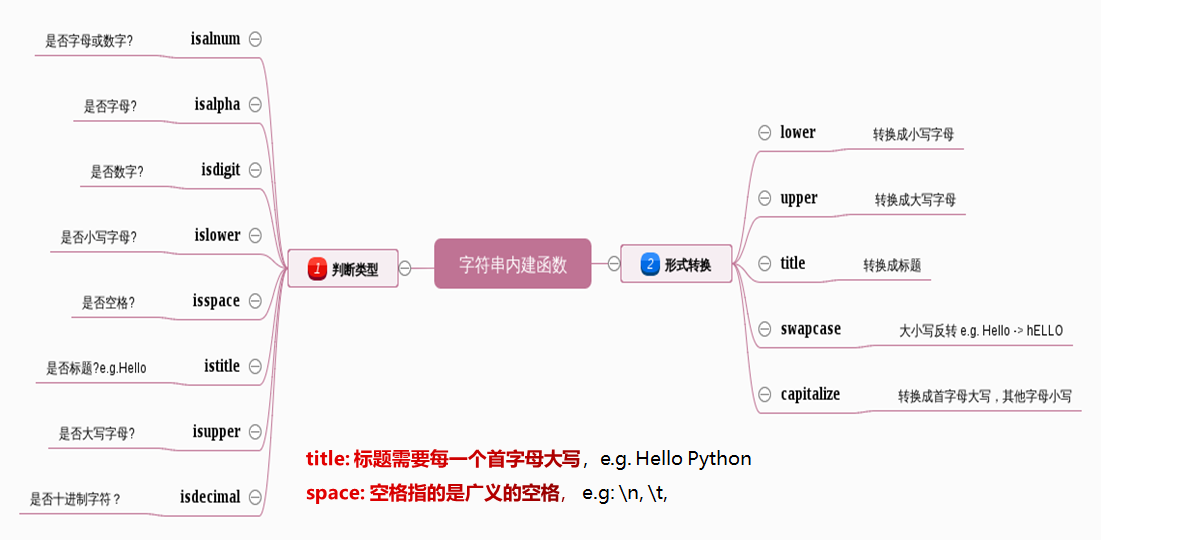## 1.字符串的判断和转换

``````s = "helloworld"
print(s.isalnum())  ##True
print(s.isdigit())  ##False
print(s.isupper())  ##False
``````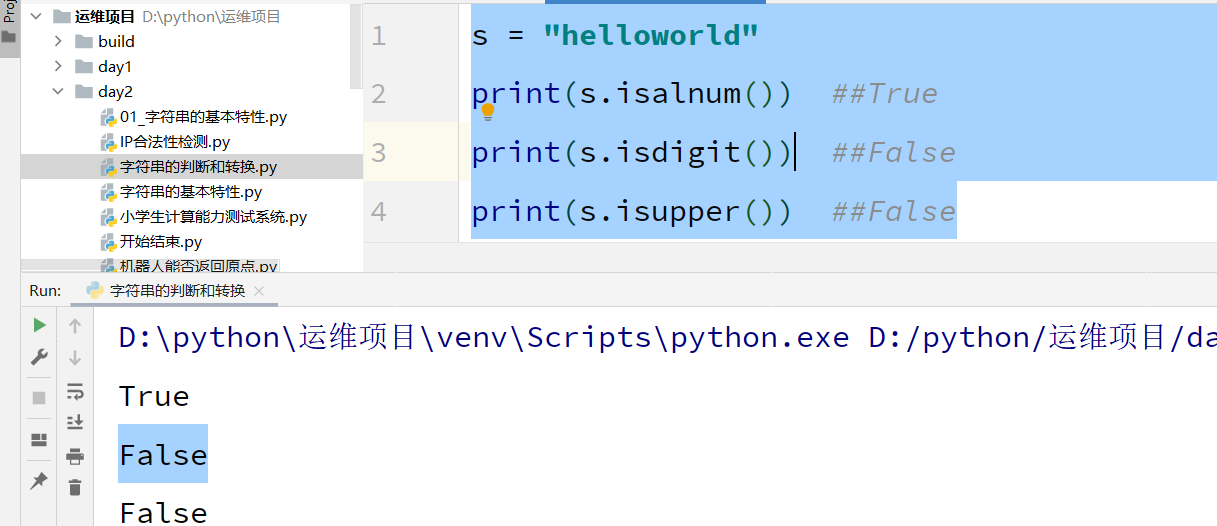## 2.类型转换

``````## 2.类型转换
print('hello'.upper())  ##HELLO
print('HELLO'.lower())   ## hello
print('HELLO world'.title())  ## Hello World
print('HELLO world'.capitalize()) ## Hello world
print('HELLO world'.swapcase())  ##hello WORLD
``````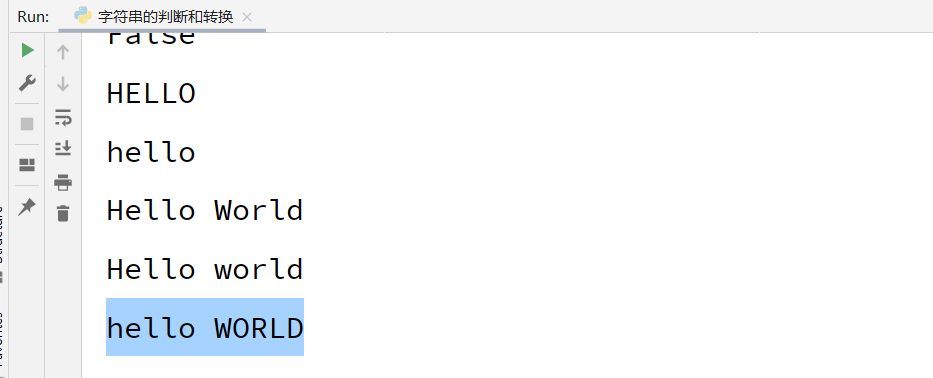``````# 需求：用户输入Y或者y都继续执行代码
# yum install httpd
choice =  input ('是否继续安装程序(y|Y):')
if choice.lower() == 'y':
print("正在安装程序.....")正在安装程序
``````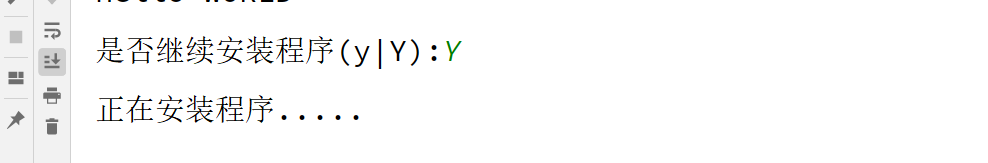## 3.字符串开头和结尾的判断``````# startswith
url = 'http://www.baidu.com'
if url.startswith('http'):
print(f'{url}是一个正确的网址，可以爬取网站')
# endswith:
# 常用场景：判断文件类型
filename = 'sun.png'
if filename.endswith('.png'):
print(f'{filename} 是图片文件')
elif filename.endswith('.mp3'):
print(f'{filename}是音乐文件')
else:
print(f'{filename}是未知文件')
``````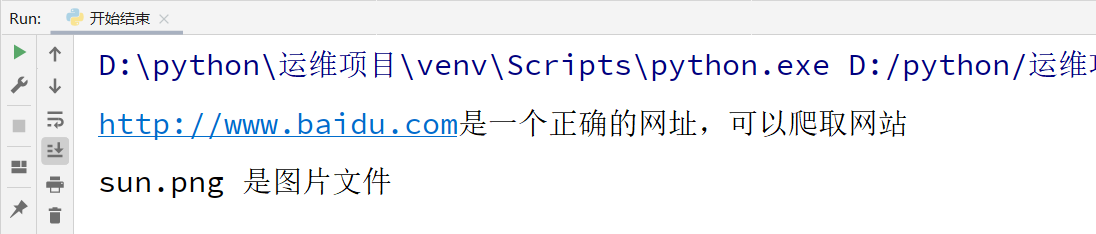#pycharm常用的快捷键:
#如何查看方法的源代码和解释说明: ctrl键按住，
#鼠标移动到你想要查看方法的位置，点击即可进入源码及方法说明

## 4.字符串的数据清洗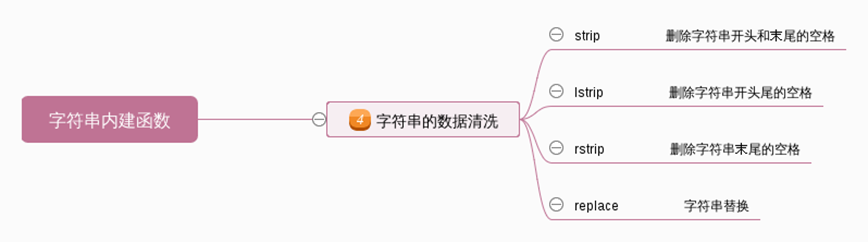• lstrip: 删除字符串左边的空格(指广义的空格: \n, \t, ’ ')
• rstrip: 删除字符串右边的空格(指广义的空格: \n, \t, ’ ')
• strip: 删除字符串左边和右边的空格(指广义的空格: \n, \t, ’ ')
• replace: 替换函数， 删除中间的空格， 将空格替换为空。replace(" ", )
``````s = ' hello'
print(s)
print(s.lstrip())
s = 'hello '
print(s.rstrip())
s = ' hello '
print(s.strip())
s = 'hel        lo'
print(s.replace(" ",""))
``````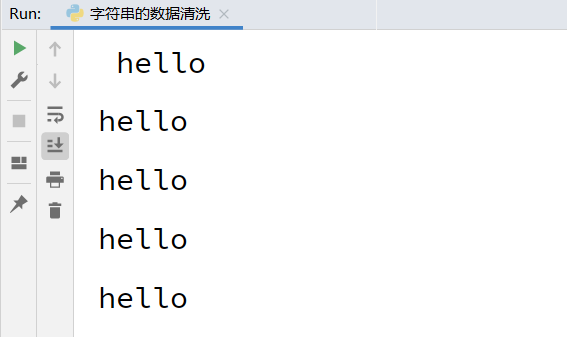## 5.字符串的位置调整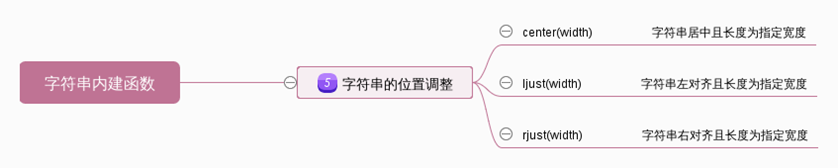``````print("学生管理系统".center(50))
print("学生管理系统".center(50,"*"))
print("学生管理系统".center(50,"-"))
print("学生管理系统".ljust(50,"*"))
print("学生管理系统".rjust(50,"*"))
``````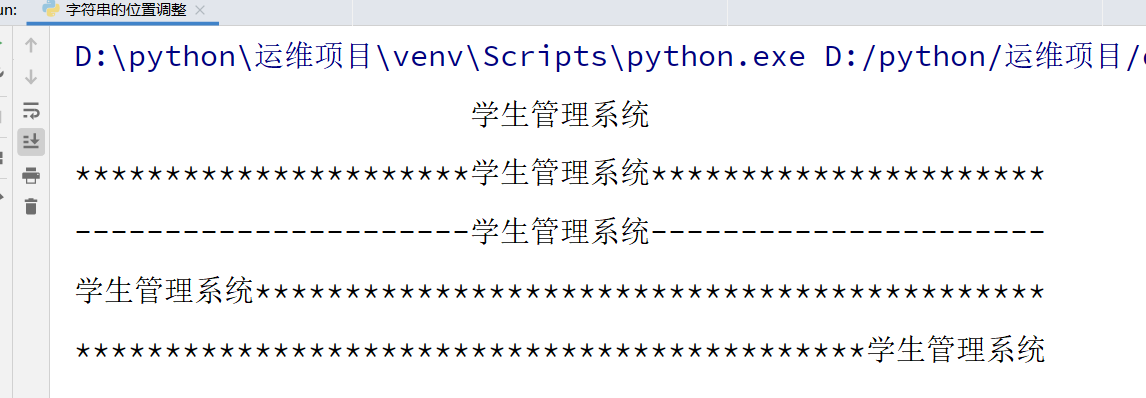## 6.字符串的搜索和统计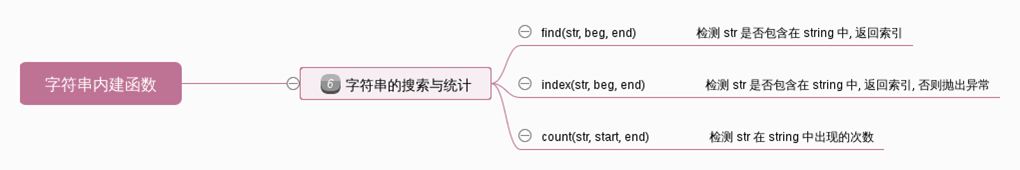``````s = "hello world"
print(s.find("llo"))
print(s.index("llo"))
print(s.find("xxx"))
print(s.index("xxx"))
# find如果找到子串， 则返回子串开始的索引位置。 否则返回-1
# index如果找到子串，则返回子串开始的索引位置。否则报错(抛出异常).
print(s.count("l"))    ## 判断字符出现次数
``````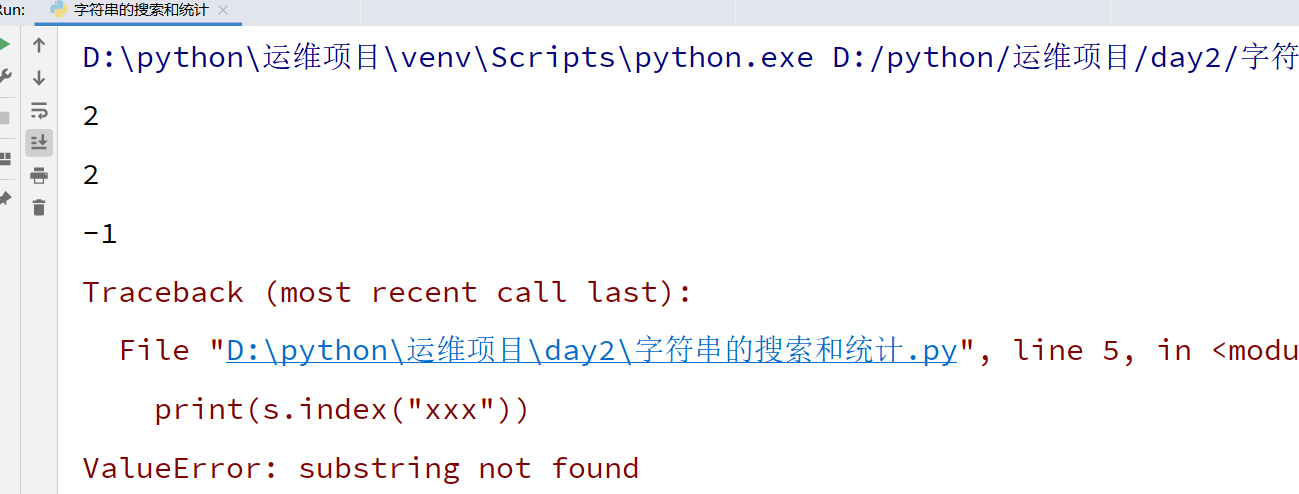## 6.字符串的分离和拼接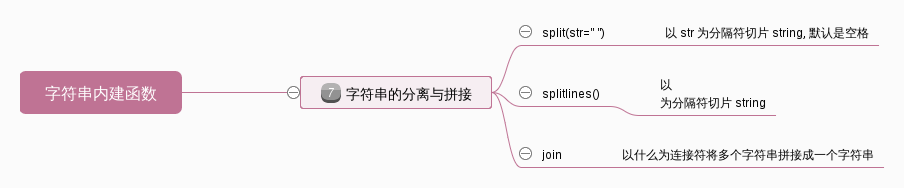``````ip = '172.25.254.100'
print(ip.split('.'))
items = ip.split('.')
print('-'.join(items))
``````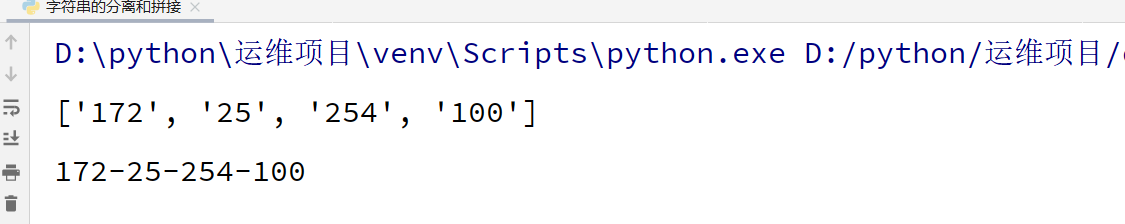# 四、拓展:string模块

``````import random
import string
print(string.digits)
print(string.ascii_letters)
print(random.sample(string.digits,2))
print(random.sample(string.ascii_letters,2))
print("".join(random.sample(string.digits,2)))
print("".join(random.sample(string.ascii_letters,2)))
``````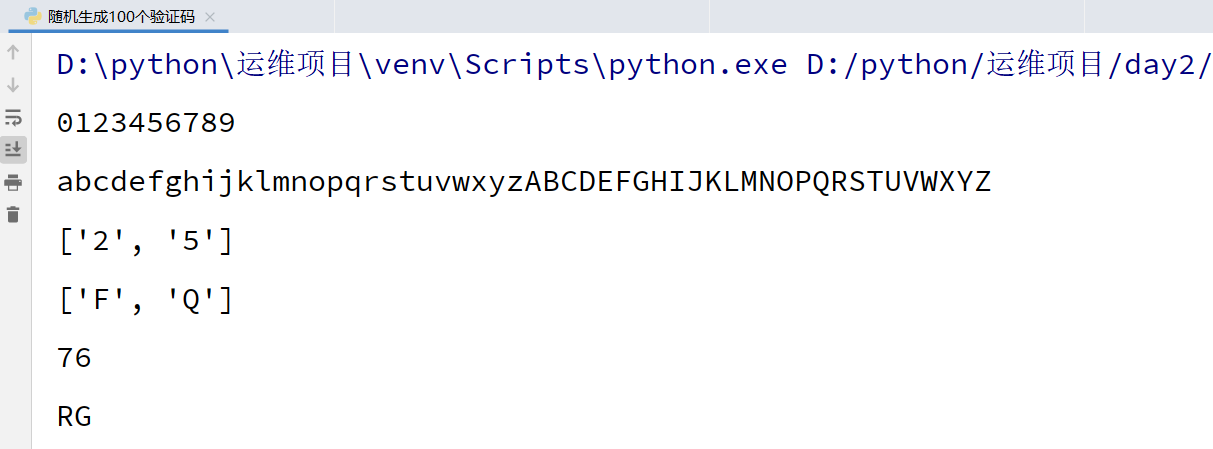``````# 需求: 生成100个验证码， 每个验证码由2个数字和2个字母组成。
for i in range(100):
print("".join(random.sample(string.digits,2)) + "".join(random.sample(string.ascii_letters,2)))
``````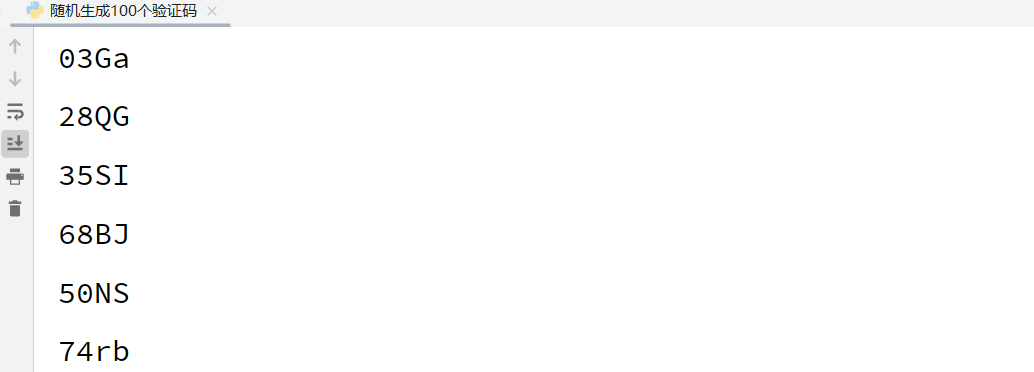# 五、练习题

## 1.IP检测

1). IPv4 地址由十进制数和点来表示，每个地址包含4个十进制数，其范围为 0 - 255， 用(".")分割。

2). IPv4 地址内的数不会以 0 开头。比如，地址 172.16.254.01 是不合法的。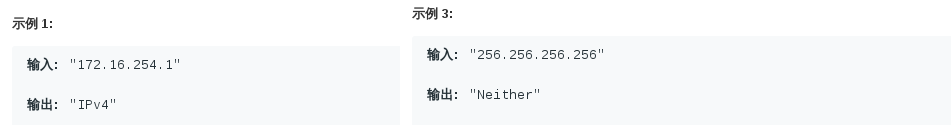## 2.小学生计算能力测试系统

``````"""
设计一个程序，用来实现帮助小学生进行算术运算练习，它具有以下功能：
提供基本算术运算(加减乘)的题目，每道题中的操作数是随机产生的，
练习者根据显示的题目输入自己的答案，
程序自动判断输入的答案是否正确并显示出相应的信息。最后显示正确率
"""
import random
count = 10
right_count = 0
for i in range(count):
num1 = random.randint(1,10)
num2 = random.randint(1,10)
symbol = random.choice(["+","-","*"])
reslt = eval (f"{num1}{symbol}{num2}")
question = (f"{num1}{symbol}{num2} = ?")
print(question)
print ("正确")
right_count +=1
else:
print("错误")
print ("正确率：%.2f%%" %(right_count/count*100))
``````

暂无相关的数据...